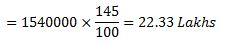## Practice Questions – Misc Aptitude – 5

1. A milkman purchases the milk at Rs. x per liter and sells it at Rs. 2x per litre still he mixes 2 liters water with every 6 liters of pure milk. What is the profit percentage?

(a) 116%

(b) 166.66%

(c) 60%

(d) 100%

(e) None of these

2. There are 20 poles with a constant distance between each pole. A car takes 24 second to reach the 12th pole. How much will it take to reach the last pole?

(a) 41.45

(b) 40.55

(c) 42.35

(d) 47

(e) None of these

3. All the page numbers from a book are added, beginning at page 1. However, one page number was mistakenly added twice. The sum obtained was 1000. Which page number was added twice?
(a) 44

(b) 45

(c) 10

(d) 12

(e) None of these

4. A milkman borrowed Rs. 2500 from two money lenders. For one loan he paid 5% p.a and for other 7% p.a. The total interest paid was Rs.275. How much did he borrow at 7% rate.

(a) Rs.600

(b) Rs.625

(c) Rs.650

(d) Rs.675

(e) None of these

5. A cistern is normally takes 6 hours to be filled by tap but it takes two hours extra because of leak. In how many more hours the leak will empty the cistern?

(a) 20 hours

(b) 24 hours

(c) 26 hours

(d) 18 hours

(e) None of these

6. In an examination pupil’s average marks were Rs.63 per paper. If he had obtained 20 more marks for his Geographic paper and 2 more marks for his history paper, his average per paper would have increased to 65. How many papers were there in the examination?

(a) 8

(b) 9

(c) 10

(d) 11

(e) None of the above

7. A man sitting on a train which is travelling at 50 kmph observes another goods train, travelling opposite direction, takes 9 sec to pass him. If the goods rain is 280 m long, find its speed?

(a) 62 kmph

(b) 58 kmph

(c) 52 kmph

(d) 50 kmph

(e) None of these

Percentage profit earned by two companies over the years 1991- 1997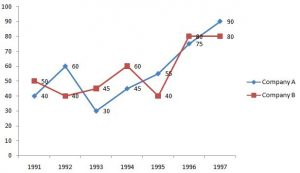8. Income of company A in 1995 was 21.7 lakhs. What was the investment?

(a) Rs.14.5 Lakhs

(b) Rs. 15.4 Lakhs

(c) Rs.15.8 Lakhs

(d) Rs. 14 Lakhs

(e) None of these

9. Income of company A in 1995 is equal to the investment of the company B in 1996. What is the ratio of the investment of company A in 1995 to the investment of company B in 1996?

(a) 31:36

(b) 31:20

(c) 20:31

(e) None of these

10. Investment of Company B in 1993 was 1540000. What was its income in that year?

(a) 23.33 Lakhs

(b) 22.33 Lakhs

(c) 22.23 Lakhs

(d) 23.23 Lakhs

(e) None of these

1. (b) 2. (a) 3. (c) 4. (b) 5. (b) 6. (d) 7. (a) 8. (d) 9. (c) 10. (b)

Explanation

1. (B) Assume that milkman purchased 6 litres of milk and one litre of milk costs 1 rupee. So CP= Rs.6

He sells it for Rs.2 then his SP= Rs.12. also he is adding 2 litre of water so 2 litre gains an amount of Rs.4.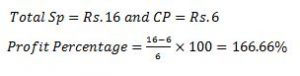2. (A)

The car starts at first pole and reaches 12th pole within 24 seconds.

To find the time taken by a car,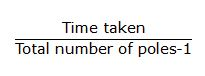Time to cross a single pole=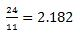Time to cross a 20 poles= 19×2.182=41.45

3. (C) We know that

Sum of natural numbers= (N(N+1))/2

We don’t know the value of N. So substitute some value and find the value of N. If we take N as 50 then the Sum will be 1275.  Sum is larger than 1000. So we can’t take it.Then go for 45 then the sum is 1035. So go for 44 then the sum will be 990.

So if we add 10 twice then the sum will be 1000. So 10 is the answer.

4. (B) Let the amount borrowed for 5% is X then

For 7% = 2500-X

Now I1+ I2= 275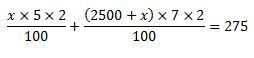By solving,

X = 1875

For 7% rate, sum = 2500-1875  = 625

5. (B)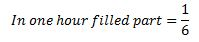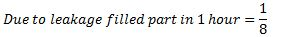Cistern fill in 6 hours.

Part of the cistern is emptied due to leakage in 1 hour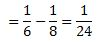So the leak can empty the cistern in 24 hours.

6. (D)

Let the number of papers be X. Then 63X+ 20 + 2 = 65X.

Then X= 11.

Alternate way.

Due to the Extra mark of 22, average increases by 2. So by dividing 22/2 we can get 11 as a answer.

7. (A) Because we are taking man as a reference there is no need to take the first train length.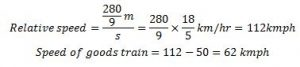8. (D)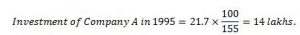9. (C) Let the income in 1995 of company A and Investment of company B in 1996 be x.

Then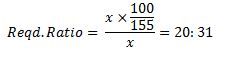10. (B)

Income of company B in 1993